Search IntMath
Close

450+ Math Lessons written by Math Professors and Teachers

5 Million+ Students Helped Each Year

1200+ Articles Written by Math Educators and Enthusiasts

Simplifying and Teaching Math for Over 23 Years

#### Tips, tricks, lessons, and tutoring to help reduce test anxiety and move to the top of the class.

By Murray Bourne, 30 May 2010

We all learn to use degrees when measuring angles, but most science and engineering applications use radians.

Radians are an alternative way of measuring angles, or the amount of turn. A lot of people get confused by radians and that's a shame, as they are not that complicated.

The definition of a radian puts a lot of people off before they even start. Here's one from MathWorld:

The radian is a unit of angular measure defined such that an angle of one radian subtended from the center of a unit circle produces an arc with arc length 1.

Say what?

Let's investigate what a radian means using a circle of radius 6. Why? Because radians work for any circle, not just the unit circle. (A unit circle has radius 1 unit).

The following circle is not an image - it's an interactive graph.

Drag the point D around this circle (with radius 6) and note what the angle is in radians. The length of the blue arc also changes as you go around the circle. Anti-clockwise is positive.


//<![CDATA[
brd = JXG.JSXGraph.initBoard('jxgbox8',
{axis:true,originX: 200, originY: 250, unitX: 30, unitY: 30,showCopyright:false});
axT1 = brd.create('text',[0.1,6.7, '&alpha; = &pi;/2 &asymp; 1.57']);
axT2 = brd.create('text',[-5.8,0.4, '&alpha; = &pi; &asymp; 3.14']);
axT3 = brd.create('text',[0.1,-6.4, '&alpha; = 3&pi;/2 &asymp; 4.17']);
var qr = [];
qr = brd.create('point', [0,0], {fixed:true,visible:false});
qr = brd.create('point', [1,0],{visible:false});
var circ = brd.create('circle',[qr, myRadius],{strokeColor:'#bfbfbf', strokeWidth:1});
gmm = brd.create('glider', [3,2.7,circ], {style:5,fillColor:'magenta',label:'P'});
ang1 = brd.create('angle', [qr,qr,gmm]);
arc1 = brd.create('arc', [qr,qr,gmm]);
var li1 = brd.createElement('segment',[qr,gmm],
{strokeWidth:2, strokeColor:'#dddddd'});
el2 = brd.create('text',[4,6.5,
function(x){
if(gmm.X()<0) {
myTheta = Math.round(100*(Math.PI+Math.atan(gmm.Y()/gmm.X())))/100;
}else if (gmm.Y() < 0){
myTheta = Math.round(100*(2*Math.PI+Math.atan(gmm.Y()/gmm.X())))/100;
} else {
myTheta = Math.round(100*Math.atan(gmm.Y()/gmm.X()))/100;
}
if (myTheta == 1) {
} else {
}
return "&alpha; = " + myTheta + units;
}
],{fontSize:16+'px'}
);
el3 = brd.create('text',[4,7.5,
function(x){
if(gmm.X()<0){
} else if (gmm.Y() < 0){
} else {
}
return "Arc length = " + myLength;
}
],{fontSize:14+'px'}
);
//]]&gt;



When you get to 1 radian, it should look like this (this is a screen shot image):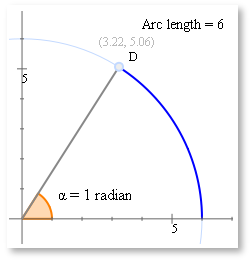Note the arc length is the same as the radius (both equal to 6 in this case).

And that's all one radian is - the angle we get when we rotate around a circle to the point where the arc length equals the radius.

Another way of putting it is that an angle measured in radians is given by: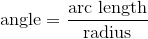In fact, here are some special angles as measured in radians: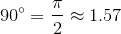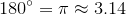and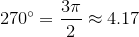#### Where do these values come from?

Let's look at the case for one complete revolution of our circle. Recall the formula for the circumference of a circle: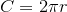Now if we go once right around the circle in the interactive graph above, the arc length (the circumference) is 2π × 6. And remember, the angle in radians is the arc length divided by the radius.

So in radians, the angle right around this circle is: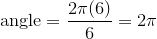Following similar thinking for 1/4 the way around the circle, 1/2 way round and 3/4 of the way around, we get the special values given above.

Note 1: For the first 2 numbers in each of the statements above, I haven't written the word "radians". If there is no degree sign, then it is assumed we are talking about radians.

Note 2: Radians can be expressed using multiples of π, or they can be written as decimals.

What a lot of students do is to translate their radian angles into degrees so it's easier to understand what is going on. That's OK, but I encourage you to try to think in radians. You'll be using them most of the time in higher level math.

## Why radians - what's wrong with degrees?

Angles are all about amounts of turn, and so they are closely related to circles. Radians are simply the amount we turn around a circle, calculated as the length of the arc we form, divided by the radius of the circle.

Many problems can be calculated directly if we know the angle in radians. For example, I can find the arc length for a particular circle by just applying the radian definition in reverse:

So for example, the circle above has radius 6, so if the angle is 2 radians (about 114°), the arc length will be:

arc length = 6 × 2 = 12 units

Try it out in the interactive graph above. When your angle is 2 radians, the arc length should be 12 units.

There are many other mathematical problems where it only "works" in radians, for example differentiation and integration problems in calculus.

## Care required

One of the most common mistakes students make after they have studied radians is to forget to check that their calculator is in the correct mode. Make sure radian mode is selected on your calculator when the question is given in radians.

## Back to the definition

I hope the definition we saw earlier makes more sense now.

The radian is a unit of angular measure defined such that an angle of one radian subtended from the center of a unit circle produces an arc with arc length 1.

The phrase "subtended from the center" just means the center of the circle is the center of our turn.

If we have a unit circle, the arc length will need to be one unit to give us one radian. If the radius of the circle is 2 units, the arc length will be 2 units.

For the example we played with above, the radius was 6 units and the arc length was 6 units to give us an angle of one radian.

## Conclusion

Radians are an alternative way of measuring angles. Once you start using them, you might wonder why we ever learned about degrees.

[The interactive graph above was made using JSXGraph.]

1. Colleen Young says:

Thank you for this - I think I'll direct my students here!
You are right - they do cause confusion and I often wonder if they should be introduced earlier.

By the time students meet radians they think that the only way angles are measured is in degrees.

2. Syed Husain says:

it is customery to write eqaution for displacement of simple harmonic motion x=A*cos(w*t+theta), here w and theta are in radians per second and degrees respectively. though it is not right to have different units but thats the way it is written in most textbooks. and there arises the problem. when a problem asked to find x for a particular time people often forget this fact. one must convert "w" either in degree or "theta" in radian, otherwise the answer will be wrong because you are adding two different units.

3. DEPR says:

4. Murray says:

@Colleen and @Depr - you are welcome. Glad you found it useful.

@Syed: Thanks for raising that issue. Yes, in some engineering contexts there is a mix of degrees and radians in the one sine expression. It's probably not such a good idea!

5. mongmara says:

It is a very interesting topic.

6. Desmond says:

Thanks for covering radians, especially the question "Why radians? Whats wrong with degrees?. Sometimes its so hard to convince my students.

7. Suprakash Chakraborty says:

Kudos 2 u,
It's really amazing 2 get the information what we've known earlier and u really explained in such a manner that Math's really an interesting subject. Myself actually wait for yr Mathsnews always and really got insight in2 very amazing facts with maths. Kudos 2 u & yr all collegues

Looking forward with long association.

8. Harmony says:

Thanks so very much!!! I've had trouble remembering to use and how to use radians in my exams, but all the while, I've NEVER known what the heck radians were. It's incredibly enlightening to finally know what radians is. I've never thought to find out what it was, it just never came up. But now that I know, I'm sure I'll never forget to use it or how to. And it's also helpful to know WHY radians instead of degrees.

9. Murray says:

@Mongmara, Desmond, Suprakash and Harmony: You're all welcome. Glad it helped!

10. P chandra sekhara rao says:

Thanks for that wonderful explanation.It is very useful for mathematics students.Why radians explantion is incredible.

11. hailegwergs says:

thanks it is very important to improve my knowledge.
and i am happy to continuous like this knowledge.

12. Someone says:

I want to know does a protractor in radians exist?

13. Murray says:

Hi. I couldn't find any real radians protractor, but here is one that will measure angles on your computer screen in both degrees and radians: Screen Protractor.

14. zizo says:

first of all thanks a lot

second i think there is a mistake in line 32

it's not 3pi/2 = 4.17 = 270 degrees

correction = 4.71 =

15. Murray says:

Hi Zizo. Thank you! This was a human coding error (by me), not a problem with JSXGraph.

I appreciate your taking the time to give constructive feedback.

16. Tim says:

Nice discussion of this topic. I'm thinking about sending my students here but, as a mathematician, I'm uncomfortable with your use of the equal sign. Pi/2 is not equal to 1.57, it is only an approximation. This may seem like picking nits, but my students often fail to understand what the symbol "=" means and misuse it.

17. Murray says:

Hi Tim. Not picking nits at all! I agree we should be more careful about approximate values and the use of "=". (But some of these issues get relegated to slightly less importance when spending ages getting the interactive graph to work, writing the text, and so on).

I have amended the post (including the interactive) - and I hope you are comfortable to send your students here now!

18. Siddhant says:

Thanks a lot...it really helped me...do you have such a page for basic calculus like integration, differentiation, limits, etc. or one for understanding solid angles?

19. Murray says:

Hi Siddhant. You can start here: Differentiation (it includes a brief explanation on limits.)

This might also be useful: Integration.

All topics can be found in the IntMath Sitemap.

All the best with your studies!

20. kulaithem says:

Thanks a lot for these beneficial information , I will look
at this topic again and try to catch it..

Thaks again=)

21. Jennifer Silverman says:

Hi all,

If you are still interested in finding protractors scaled in radians, you can find them at my website, http://www.proradian.net. After 14 years of teaching, and jumping through hoops to get kids to grasp radians, I developed a new pair of protractors and am having them made. My selling price is less than my cost at this point, but I think they're a great tool. (And I agree - start in geometry! The pattern for interior angles of polygons, for example, is a cake-walk in terms of pi.)

Sincerely,

Jennifer Silverman

22. Wright says:

Thank you- despite my abilities as a teacher - this is an elusive topic for many kids. This comes about (I suppose) of having only worked in degrees and then, told nothing of their history.
I ask students: "Why 360o. in a circle?" They say: "because a right angle has 90 degrees!"
See my point?

### Comment Preview

HTML: You can use simple tags like <b>, <a href="...">, etc.

To enter math, you can can either:

1. Use simple calculator-like input in the following format (surround your math in backticks, or qq on tablet or phone):
a^2 = sqrt(b^2 + c^2)
(See more on ASCIIMath syntax); or
2. Use simple LaTeX in the following format. Surround your math with $$ and $$.
$$\int g dx = \sqrt{\frac{a}{b}}$$
(This is standard simple LaTeX.)

NOTE: You can mix both types of math entry in your comment.